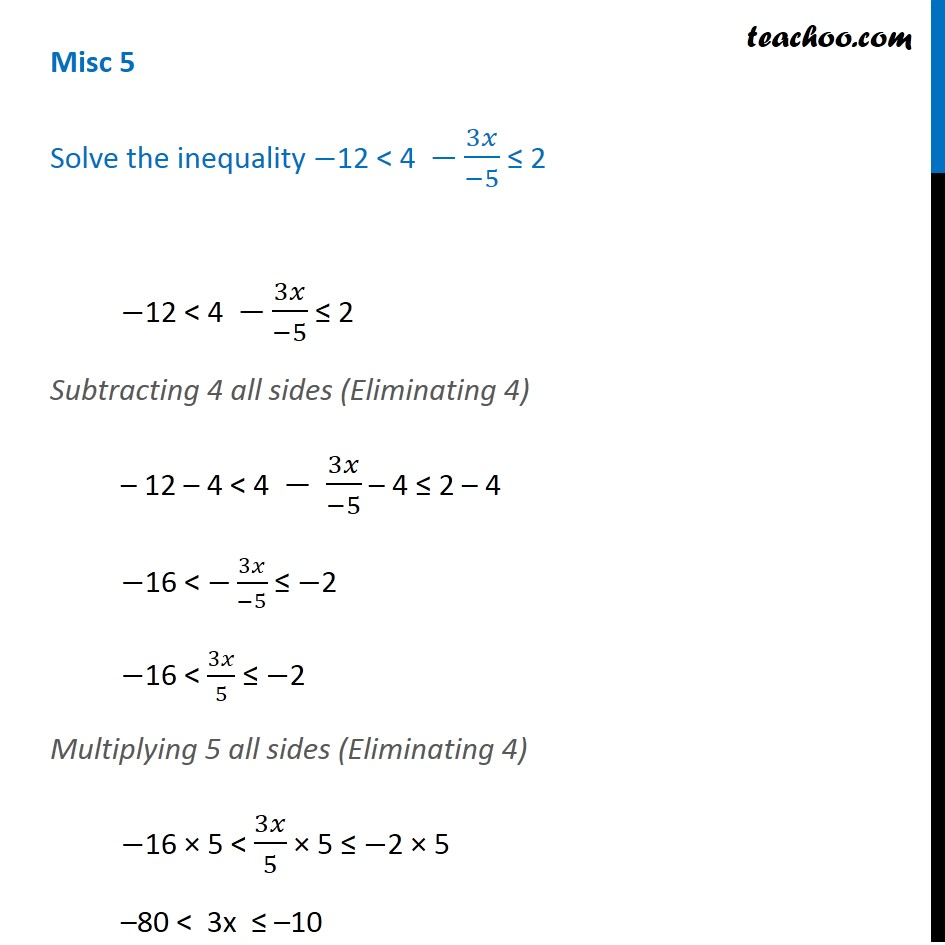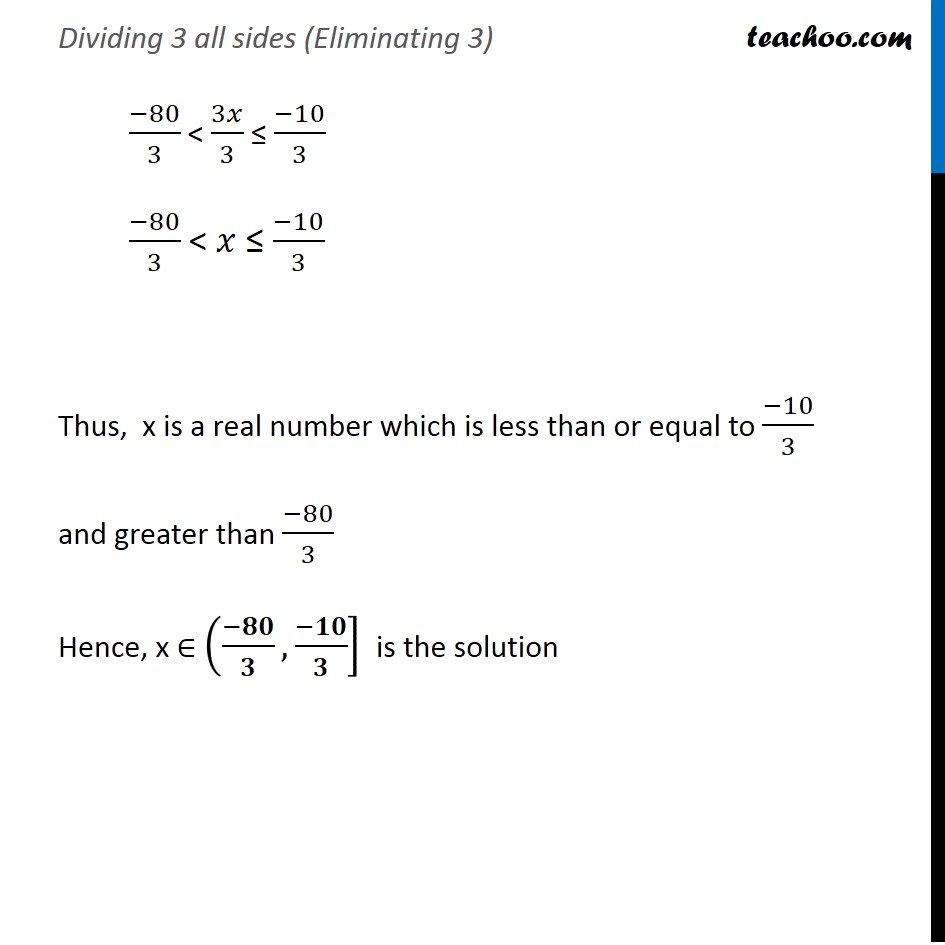Miscellaneous

Chapter 5 Class 11 Linear Inequalities
Serial order wiseLearn in your speed, with individual attention - Teachoo Maths 1-on-1 Class

### Transcript

Misc 5 Solve the inequality −12 < 4 −3𝑥/(−5) ≤ 2 −12 < 4 −3𝑥/(−5) ≤ 2 Subtracting 4 all sides (Eliminating 4) – 12 – 4 < 4 − 3𝑥/(−5) – 4 ≤ 2 – 4 −16 < −3𝑥/(−5) ≤ −2 −16 < 3𝑥/5 ≤ −2 Multiplying 5 all sides (Eliminating 4) −16 × 5 < 3𝑥/5 × 5 ≤ −2 × 5 –80 < 3x ≤ –10 Dividing 3 all sides (Eliminating 3) (−80)/3 < 3𝑥/3 ≤ (−10)/3 (−80)/3 < 𝑥 ≤ (−10)/3 Thus, x is a real number which is less than or equal to (−10)/3 and greater than (−80)/3 Hence, x ∈ ((−𝟖𝟎)/𝟑,(−𝟏𝟎)/𝟑] is the solution